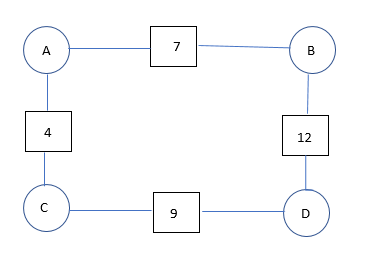# Intrigue Year 7 with their Experience of Algebra – Part 2Theme 1: Algebraic relationships: generalising from arithmetic. We believe that gaining insight into the algebraic relationships that underpin number will help pupils understand how to construct and transform algebraic expressions and equations.

Arithmagons are one of the early activities – these are problems involving patterns and relationships in numbers. They are a good introduction to writing equations. The number in each small square is the sum of the two numbers in the circles on each side of it.

Pupils find and record possible values of A, B, C and D. Ask: How many solutions can you find? Try to make your record well-organised. Check and discuss with another pair. What do you notice about the links between the values of A, B, C and D? Do they apply to each set of solutions? Record what you notice about these relationships – can you write them as equations? Find as many as you can!